Home MATHEMATICS TOPIC 1: COORDINATE GEOMETRY ~ MATHEMATICS FORM 4

# TOPIC 1: COORDINATE GEOMETRY ~ MATHEMATICS FORM 4

4701
553Equation of a Line
The General Equation of a Straight Line
Derive the general equation of a straight line
COORDINATES OF A POINT
•The coordinates of a points – are the values of x and y enclosed by the brackets which are used to describe the position of point in a line in the plane.
The plane is called xy-plane and it has two axis.
1. horizontal axis known as axis and
2. vertical axis known as axis
Consider the xy-plane below
The coordinates of points A, B, C ,D and E are A(2, 3), B(4, 4), C(-3, -1), D(2, -4) and E(1, 0).
Definition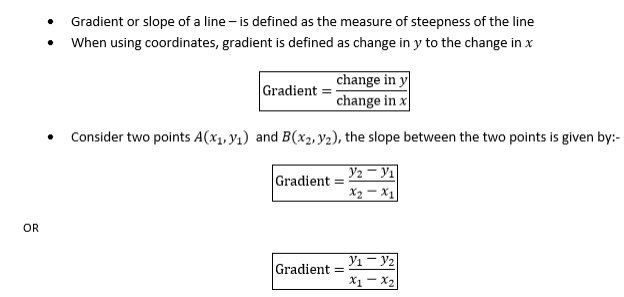Example 1
Find the gradient of the lines joining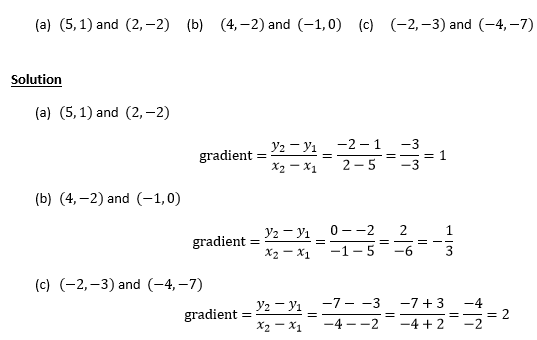Example 2
(a) The line joining (2, -3) and (k, 5) has a gradient -2. Find k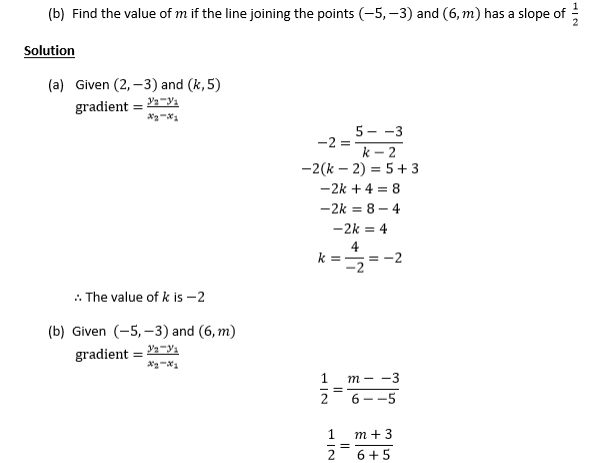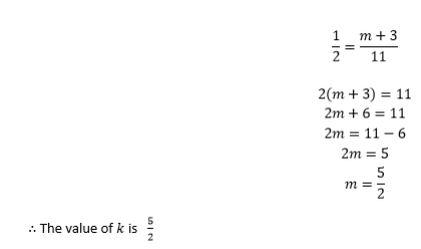Exercise 1
1. Find the gradientof the line which passes through the following points ;
1. (3,6) and (-2,8)
2. (0,6) and (99,-12)
3. (4,5)and (5,4)
2. A line passes through (3, a) and (4, -2), what is the value of a if the slope of the line is 4?
3. The gradient of the linewhich goes through (4,3) and (-5,k) is 2. Find the value of k.
FINDING THE EQUATION OF A STRAIGHT LINE
The equation of a straight line can be determined if one of the following is given:-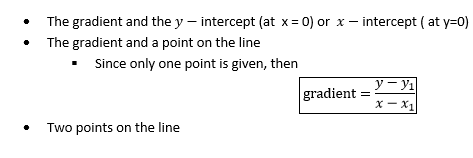Example 3
Find the equation of the line with the following
2. Gradient and passing through the point
3. Passing through the points and
Solution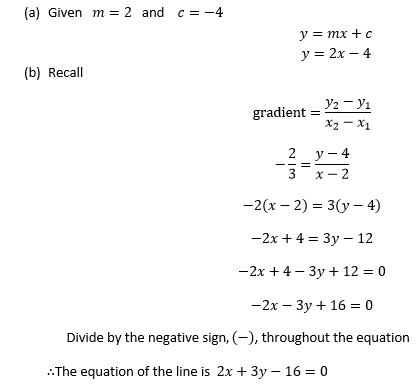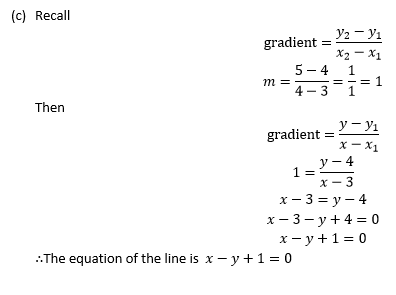EQUATION OF A STRAIGHT LINE IN DIFFERENT FORMS
The equation of a line can be expressed in two forms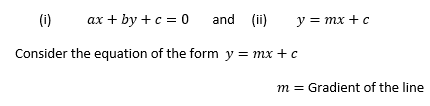Example 4
Find the gradient of the following lines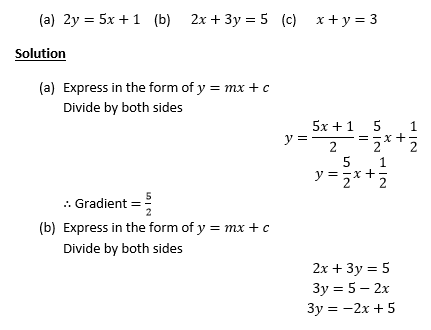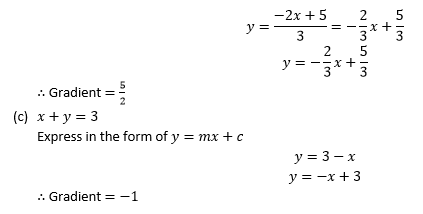INTERCEPTS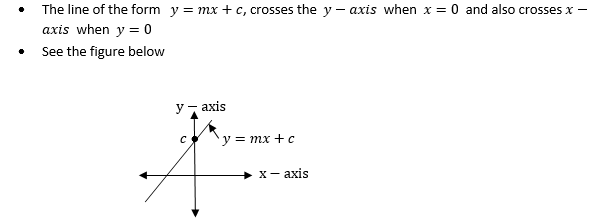Therefore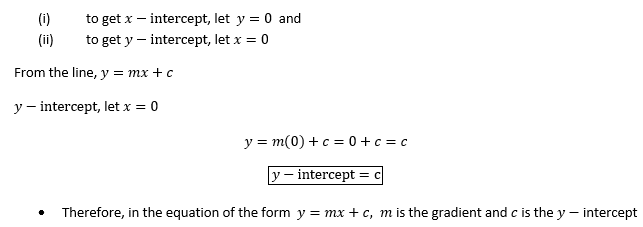Example 5
Find the y-intercept of the following lines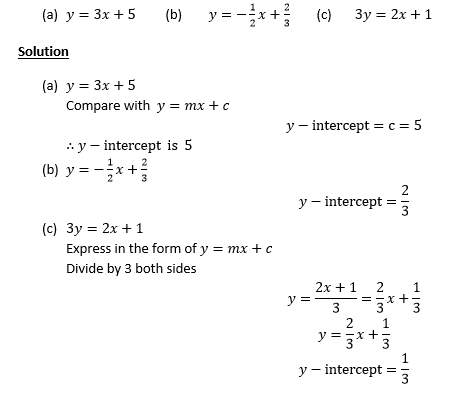Example 6
Find the x and y-intercept of the following lines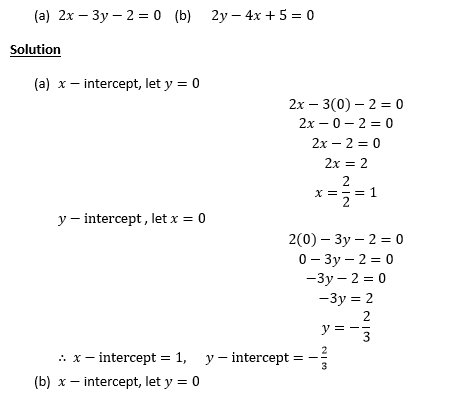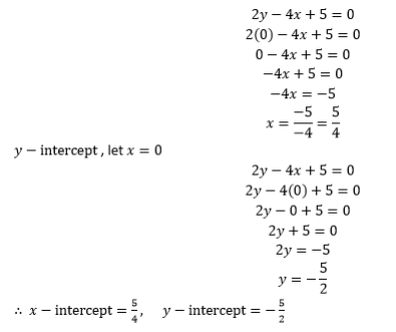Exercise 2
Attempt the following Questions.
1. Find the y-intercept of the line 3x+2y = 18 .
2. What is the x-intercept of the line passing through (3,3) and (-4,9)?
3. Calculate the slope of the line given by the equation x-3y= 9
4. Find the equation of the straight line with a slope -4 and passing through the point (0,0).
5. Find the equation of the straight line with y-intercept 5 and passing through the point (-4,8).
GRAPHS OF STRAIGHT LINES
The graph of straight line can be drawn by using the following methods;
1. By using intercepts
2. By using the table of values
Example 7
Sketch the graph of Y = 2X – 1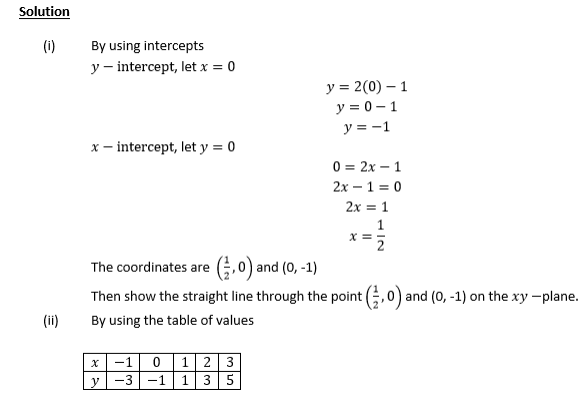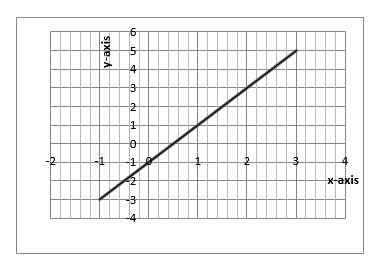SOLVING SIMULTANEOUS EQUATION BY GRAPHICAL METHOD
• Use the intercepts to plot the straight lines of the simultaneous equations
• The point where the two lines cross each other is the solution to the simultaneous equations
Example 8
Solve the following simultaneous equations by graphical methodExercise 3
1. Draw the line 4x-2y=7 and 3x+y=7 on the same axis and hence determine their intersection point
2. Find the solutionfor each pair the following simultaneous equations by graphical method;
1. y-x = 3 and 2x+y = 9
2. 3x- 4y=-1 and x+y = 2
3. x = 8 and 2x-3y = 10

1. like this blog very much its a ratting nice situation to

Do you have any? Please allow me recognize so that I may subscribe.

Thanks.

Also visit my web site :: sky1388 (sg918kiss.com)

3. It’s truly very difficult in this full of activity life to listen news on TV, therefore I simply use web for that
purpose, and get the newest news.

4. I really like what you guys are up too. This sort of clever work
and reporting! Keep up the very good works guys I’ve added you guys to my blogroll.

5. Hola! I’ve been reading your website for a long time now
and finally got the bravery to go ahead and give you
a shout out from Porter Texas! Just wanted to mention keep up the excellent job!

6. Thanks for sharing your info. I really appreciate your efforts and I will
be waiting for your next post thanks once again.

7. Please let me know if you’re looking for a writer for your weblog.

You have some really great posts and I believe I would be a good asset.
If you ever want to take some of the load off, I’d love to write some content for your blog in exchange for a link back to mine.

Please send me an e-mail if interested. Thank you!

8. Have you ever considered publishing an e-book or guest authoring on other websites?
I have a blog based on the same ideas you discuss and would love to
have you share some stories/information. I know my viewers would appreciate your work.
If you’re even remotely interested, feel free to send me an email.